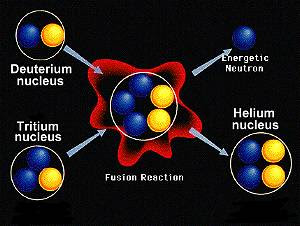# What is the difference between fission and fusion?

Aug 16, 2014

Nuclear fission is a process in which a nucleus splits into two smaller nuclei. For example,

$\text{_92^235"U" + _0^1"n" → _56^142"Ba" + _36^91"Kr" +3_0^1"n}$Nuclear fusion is a process in which two nuclei join to form a larger nucleus. For example,

$\text{_5^10"B" + _2^4"He" → _7^13"N" + _0^1"n}$